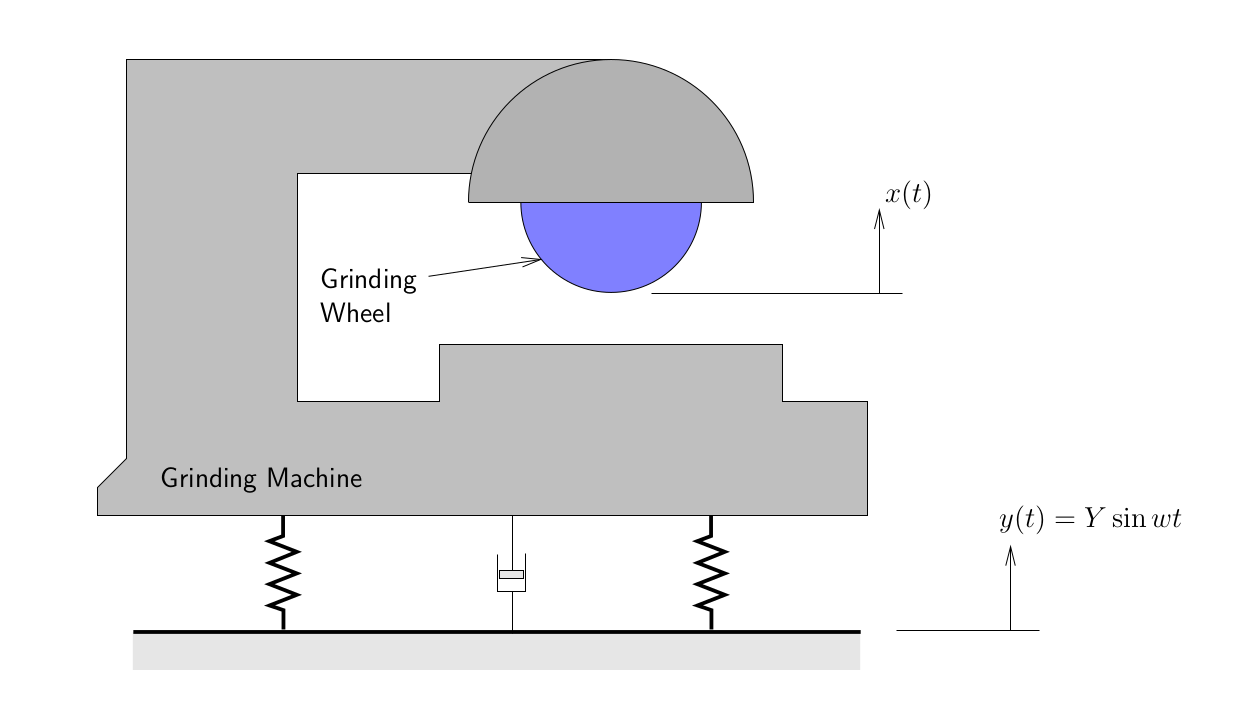### Forced Vibrations of Damped Single Degree of Freedom Systems: Chapter 5 examples

### Example 5-1

A machine with a mass of 100~kg is supported on springs of total stiffness 700~kN/m and has an unbalanced rotating element which results in a disturbing force of 350~N at a speed of 3000~RPM. Assuming the system has a damping ratio of $\zeta=0.20$, determine

1. item the amplitude of the motion due to the rotating imbalance,
2. item the transmissibility, and
3. item the maximum force transmitted to the supporting structure.

### Example 5-2

A vibrating system, together with its inertia base, is originally mounted on a set of springs. The operating frequency of the machine is 230~RPM. The system weighs 1000~N and has an effective spring modulus 4000~N/m. Shock absorbers are to be added to the system to reduce the transmissibility at resonance to 3. Additionally, the transmissibility at the normal operating speed should be kept below 0.2.

1. Without damping, what is the transmissibility at the operating speed?
2. Is it possible to pick a damper which satisfies the above conditions? If so, what range of values of the damping ratio ($\zeta$) will satisfy these two conditions?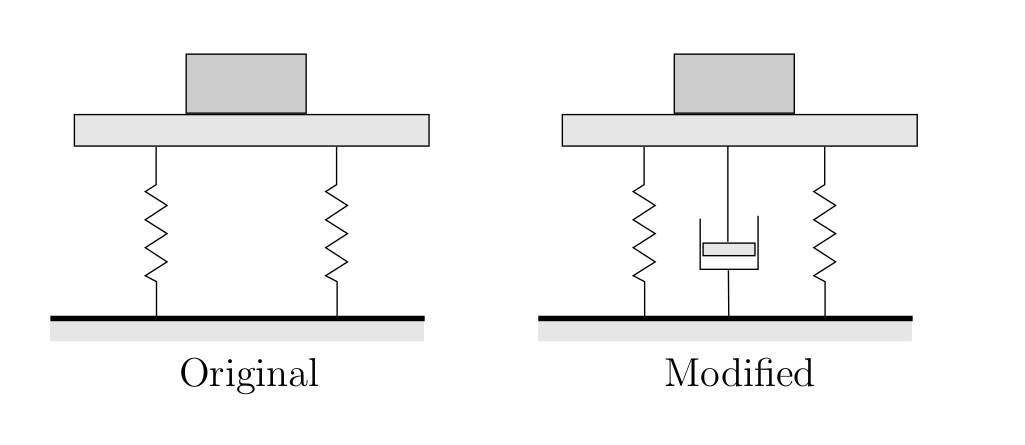### Example 5-3

A counter-rotating eccentric weight exciter is used to produce the forced oscillation of a spring and damper supported mass as shown below. By varying the rotation speed, a resonant amplitude of 0.60 cm was recorded. When the speed of rotation was increased considerably beyond the natural frequency, the amplitude appeared to approach a constant value of 0.08 cm.

Determine the damping ratio for the system.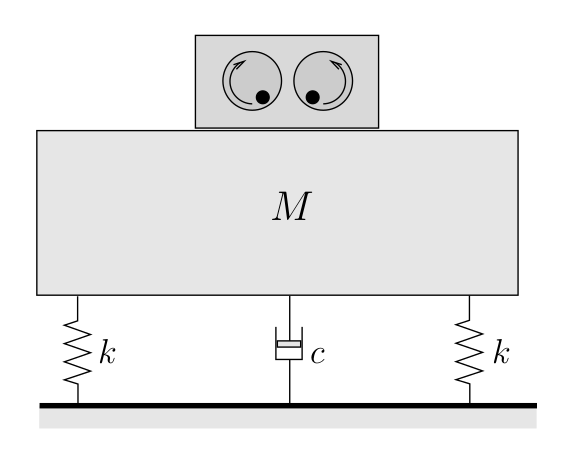### Example 5-4

An instrument package of mass $m$ is to be isolated from a vibrating surface which is moving with an amplitude $Y$ at a frequency of 600 RPM.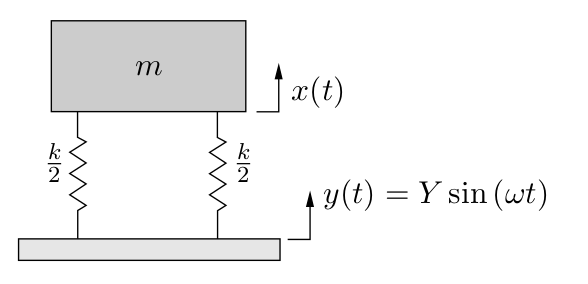1. What should the natural frequency of the system be if the maximum amplitude of the instrument package is to be $\frac{1}{8}$ of $Y$?
2. In order to reduce the amplitude of oscillation even further, several ideas (illustrated below) are proposed to improve the situation. Evaluate each of these ideas and determine if it will improve or worsen the situation. Use a combination of calculations and diagrams to support your reasoning.
1. A damper is added between the mass and vibrating surface which provides a damping ratio of $/zeta = 1.0$
2. An inertia mass equal to $3m$ is added on the same supports.
3. A damper is added between the instrument package and a fixed support to provide a damping ratio of $/zeta = 1.0$. (Note: start from the differential equation of motion.)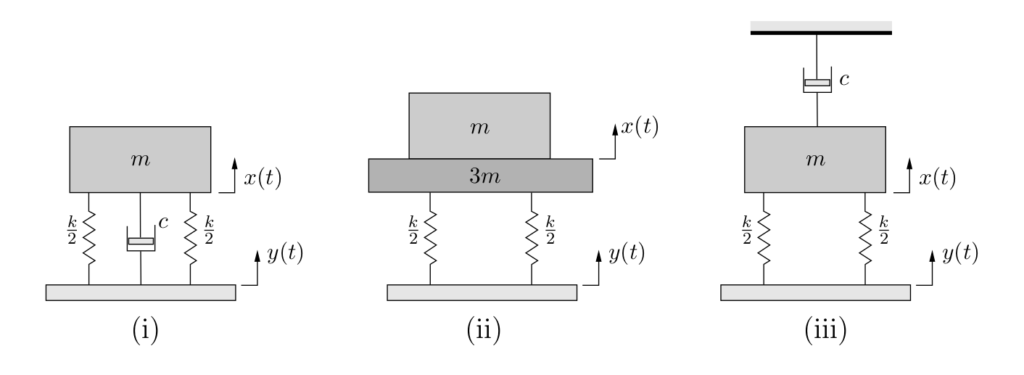### Example 5-5

A precision grinding machine is supported on an isolator that has a stiffness of 1 MN/m and a viscous damping constant of 1 kN·s/m. The floor on which the machine is mounted is subjected to a harmonic disturbing force due to the operation of a unbalanced engine in the vicinity of the grinding machine operating at a speed of 6000 RPM. Determine the maximum acceptable amplitude of the floor motion ($Y$) if the resulting amplitude of vibration of the grinding wheel is restricted to be 10 −6 m. Assume that the grinding machine and the wheel are a rigid body of weight 5000 N.